Browse by Topic
Related Topics

# Interactive Pythagoras Trees with webMathematica 3

I wanted to build a simple web application for manipulating and exporting Pythagoras trees to make posters and desktop wallpaper, and so I turned to the new features of webMathematica 3.

webMathematica is a web application framework released by Wolfram Research. It allows users to write web pages using Mathematica, seamlessly integrating Mathematica code with HTML and JavaScript.

webMathematica 3, the new version released on September 15, introduces several new features such as a web version of the popular Manipulate command and a way to evaluate Mathematica code asynchronously, without delaying page loading.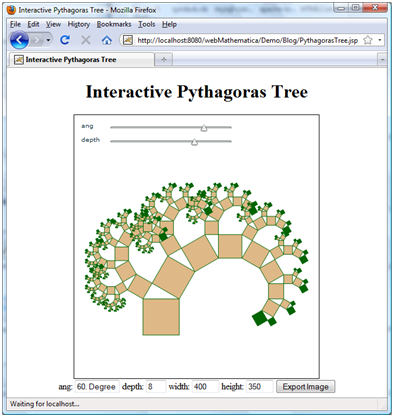What are Pythagoras trees, and how can we use webMathematica 3 to explore them?

A Pythagoras tree is a fractal constructed by successively adding squares to the sides of right triangles, where the two leaves of one tree then become the bases for subtrees.

Here is a function, written by Maxim Rytin, for generating a tree with a certain angle between sides and a certain number of subtrees.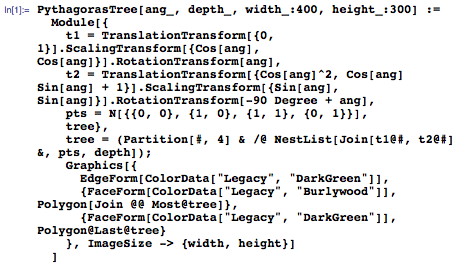Mathematica allows me to generate Pythagoras trees and combine geometric transforms, list manipulation, and graphics constructs in just a few lines of code.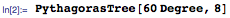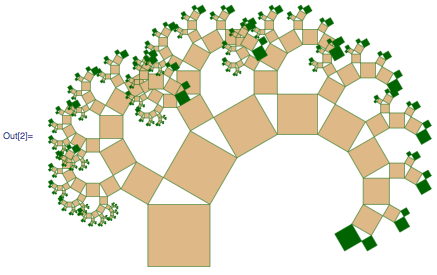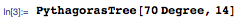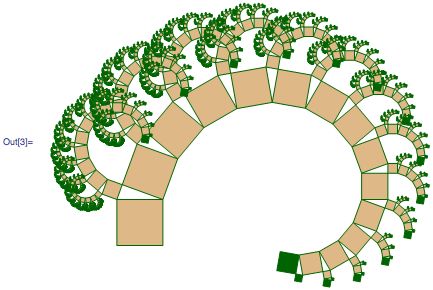To manipulate the angle and depth in a notebook, you would use it like this: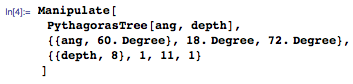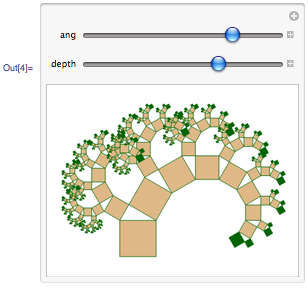The ang variable controls the two remaining angles of the right triangles, and the depth variable controls the number of subtrees.

But let’s say that you want to provide the power of Manipulate to users from inside a web page. You may want to incorporate the Manipulate into a larger website, or display data or models that would not be possible on the desktop.

How would you do it?

Here is example source code for an entire web page that uses the web version of Manipulate from within webMathematica:

```<%@ taglib uri="http://www.wolfram.com/msp" prefix="msp" %>

<msp:evaluate>
Needs["MSPManipulate`"];
Get[ToFileName[{MSPPageDirectory[]}, "PythagorasTree.m"]]
</msp:evaluate>

<html>
<head>
<title>Interactive Pythagoreas Tree</title>
<meta http-equiv="Content-Type" content="text/html; charset=iso-8859-1"/>
<link rel="stylesheet" type="text/css" href="Pythagoreas.css" />
<msp:evaluate>
MSPManipulateHeader[\$\$updateArgs, \$\$manipulateNumber]
</msp:evaluate>
</head>

<body>

<div class="content">

<h1>Interactive Pythagoreas Tree</h1>

<msp:evaluate>
MSPManipulate[
PythagorasTree[ang, depth],
{{ang, 60. Degree}, 18. Degree, 72. Degree},
{{depth, 8}, 1, 11, 1}
]
</msp:evaluate>

</div>

</body>
</html>
```

Let me explain what is going on.

webMathematica uses JavaServlet and JSP technology to provide server-side scripting functionality. With a JSP, you can write a regular HTML file, embedded with commands that run on the server before reaching the client. This can be Mathematica code as well as Java code.

Throughout the page, there are HTML tags, but there are also msp:evaluate tags. msp:evaluate tags are how Mathematica code is run inside of web pages!

The lines

```<msp:evaluate>
Needs["MSPManipulate`"];
Get[ToFileName[{MSPPageDirectory[]}, "PythagorasTree.m"]]
</msp:evaluate>
```

are an example of embedding Mathematica code into a JSP. Any content inside of msp:evaluate tags is evaluated on the server by a Mathematica kernel. In this example, the code is loading files for the Manipulate and the PythagorasTree implementations.

The lines

```<msp:evaluate>
MSPManipulate[
PythagorasTree[ang, depth],
{{ang, 60. Degree}, 18. Degree, 72. Degree},
{{depth, 8}, 1, 11, 1}
]
</msp:evaluate>
```

are where the magic happens. Inside of the msp:evaluate tags, the MSPManipulate generates code to display the Manipulate widget in the page. Notice that the syntax for MSPManipulate on the web is identical to the syntax of Manipulate in a notebook.

The code that MSPManipulate generates is rendered in a web browser like this: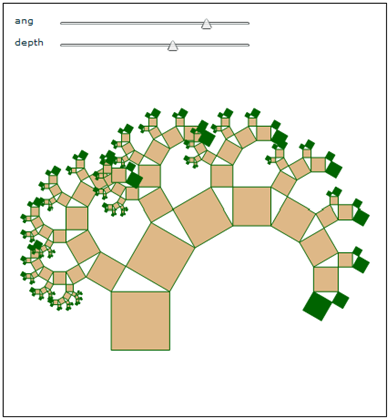The sliders can be moved around in a web browser just like Manipulate sliders can in a notebook. This is accomplished with Flash technology, the same technology that popular sites like the Wolfram Demonstrations Project and YouTube use for their players.

After I had the Manipulate working, I was happy, but I quickly ran into a roadblock.

I wanted to be able to export images to files, with control over the dimensions of the images. So some kind of additional step was needed.

Also, this implementation of PythagorasTree starts slowing dramatically at greater depths because of the very large number of graphics primitives being processed. It would be possible to implement a more efficient version, but I was willing to wait; I just didn’t want my site to look like it was hanging while the computation progressed.

Luckily, webMathematica provided the answer.

webMathematica 3 introduces a new way for evaluating expressions, but queues them so that the page does not wait for the result.

The msp:evaluateQueued tag is like the msp:evaluate tag except that it returns immediately, submits a job for the computation, and runs the job asynchronously.

I could use the msp:evaluateQueued tag to run the job in background, and check back on it when it has progressed.

First, let’s construct an HTML form to submit the job:

```<form action="PythagorasTree.jsp" method="post">
ang: <input type="text" name="ang" value="60. Degree" size="8"/>
depth: <input type="text" name="depth" value="8" size="1"/>
width: <input type="text" name="width" value="400" size="3"/>
height: <input type="text" name="height" value="350" size="3"/>
<input type="submit" name="export" value="Export Image"/>
</form>
```

Next, the msp:evaluateQueued tag is used to queue a job. The job’s id is stored in a page variable that can be checked later.

```<msp:evaluateQueued pool="Demo" var="id">
tree = PythagorasTree[MSPToExpression[\$\$ang], MSPToExpression[\$\$depth],
MSPToExpression[\$\$width], MSPToExpression[\$\$height]];
MSPShow[tree]
</msp:evaluateQueued>
```

MSPToExpression is a function that converts web input into Mathematica expressions. This allows users to specify Mathematica code as inputs, like 60 Degree for the ang parameter, or 2^4-1 for the depth parameter. This generality is not present in other server-side scripting systems, where the inputs would have to be literal numeric values.

Using the JSTL tag library—which provides several standard programming constructs for JSPs, such as loops and conditional branching—we can check the state of the job and act accordingly:

```<c:choose>

<c:when test="\${job.state == State.QUEUED}">
<p>The rendering job is queued.</p>
<p><a href="PythagorasTree.jsp?id=\${param.id}">Check again?</a></p>
</c:when>

<c:when test="\${job.state == State.RUNNING}">
<p>The rendering job is running.</p>
<p><a href="PythagorasTree.jsp?id=\${param.id}">Check again?</a></p>
</c:when>

<c:when test="\${job.state == State.TERMINATED}">
<p>The rendering job is finished.</p>
<p>Here is the result:</p>
<div>\${job.output}</div>
<p><a href="PythagorasTree.jsp">Start over?</a></p>
</c:when>

</c:choose>
```

When the job is finished, it returns a page that looks like this: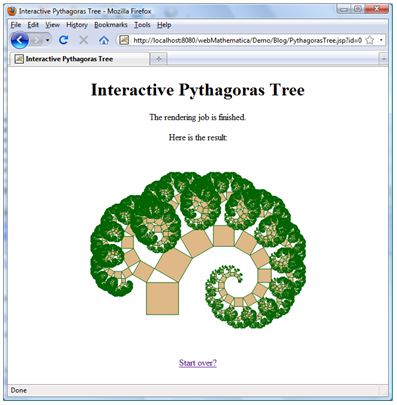I can now give people a link to my web application and let them manipulate and export Pythagoras trees, without them needing a copy of Mathematica or Mathematica Player to see the results. All they need is a web browser.

Using webMathematica 3’s new features for interactivity and asynchronous computation alongside standard JSP technology for server-side scripting allowed me to write my web application quickly and painlessly.

Even though I used webMathematica just to build this simple, single-page web application, it is also the core technology behind big sites like Wolfram|Alpha and the Integrator—it builds commercial applications as easily as simple ones.

Want to learn more about webMathematica, and webMathematica 3’s new features? Sign up for the free online “S22: Overview of webMathematica” seminar.